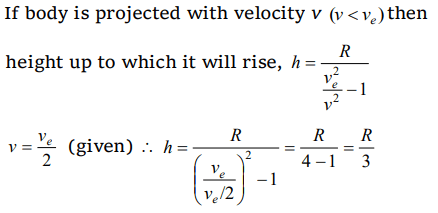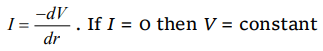## Gravitation Questions and Answers Part-13

1.A body of mass m kg. starts falling from a point 2R above the Earth’s surface. Its kinetic energy when it has fallen to a point ‘R’ above the Earth’s surface [R-Radius of Earth, M-Mass of Earth, G-Gravitational Constant]
a) $\frac{1}{2}\frac{GMm}{R}$
b) $\frac{1}{6}\frac{GMm}{R}$
c) $\frac{2}{3}\frac{GMm}{R}$
d) $\frac{1}{3}\frac{GMm}{R}$

Explanation:2. A body is projected vertically upwards from the surface of a planet of radius R with a velocity equal to half the escape velocity for that planet. The maximum height attained by the body is
a) R/3
b) R/2
c) R/4
d) R/5

Explanation:3. Energy required to move a body of mass m from an orbit of radius 2R to 3R is
a) $GMm\diagup 12R^{2}$
b) $GMm\diagup 3R^{2}$
c) $GMm\diagup 8R$
d) $GMm\diagup 6R$

Explanation:4.The kinetic energy needed to project a body of mass m from the earth surface (radius R) to infinity is
a) mgR/2
b) 2 mgR
c) mgR
d) mgR/4

Explanation:5. Radius of orbit of satellite of earth is R. Its kinetic energy is proportional to
a) $\frac{1}{R}$
b) $\frac{1}{\sqrt{R}}$
c) R
d) $\frac{1}{R^{3/2}}$

Explanation:6. In some region, the gravitational field is zero. The gravitational potential in this region
a) Must be variable
b) Must be constant
c) Cannot be zero
d) Must be zero

Explanation:7. A particle falls towards earth from infinity. It’s velocity on reaching the earth would be
a) Infinity
b) $\sqrt{2gR}$
c) $2\sqrt{gR}$
d) Zero

Explanation:8. Gas escapes from the surface of a planet because it acquires an escape velocity. The escape velocity will depend on which of the following factors :
I. Mass of the planet
II. Mass of the particle escaping
III. Temperature of the planet
Select the correct answer from the codes given below :
a) I and II
b) II and IV
c) I and IV
d) I, III and IV

Explanation:9. $V_{e}$ and $V_{p}$ denotes the escape velocity from the earth and another planet having twice the radius and the same mean density as the earth. Then
a) $V_{e}=V_{p}$
b) $V_{e}=V_{p}\diagup 2$
c) $V_{e}= 2V_{p}$
d) $V_{e}=V_{p}\diagup 4$a) $\sqrt{\frac{2GM}{R}}$
b) 2$\sqrt{\frac{GM}{R}}$
c) $\sqrt{\frac{2GMm}{R}}$
d) $\sqrt{\frac{GM}{R}}$